Study Guide

# Inverse Functions

## Inverse Functions

Inverse trig functions are sort of like bizarro trig functions. They probably were meant to live in a parallel universe, but somehow they ended up here. Now we're stuck with them. We guess we should learn how to handle them since they're here to stay.

Let's go over their notation first. Inverse trig functions are indicated with an exponent of -1.

• Inverse sine is written sin-1 x.
• Inverse cosine is written cos-1 x.
• Inverse tangent is written tan-1 x.

Similarly,

• Inverse cosecant is written csc-1 x.
• Inverse secant is written sec-1 x.
• Inverse cotangent is written cot-1 x.

This "-1" is not a true exponent. It's just a convenient way to indicate that we're using the inverse functions. We told you they were bizarre.

Just to be clear,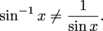You may have seen another notation for inverse functions using the prefix "arc."

arcsin x = sin-1 x
arccos x = cos-1 x
arctan x = tan-1 x
arccsc x = csc-1 x
arcsec x = sec-1 x
arccot x = cot-1 x

When working with inverse trig functions, here's how it works:

sin ɵ = x and ɵ = sin-1 x
cos ɵ = x and ɵ = cos-1 x
tan ɵ = x and ɵ = tan-1 x
csc ɵ = x and ɵ = csc-1 x
sec ɵ = x and ɵ = sec-1 x
cot ɵ = x and ɵ = cot-1 x

The normal trig functions give us a number when we plug in an angle, but the inverse trig functions spit out an angle when we plug in a number.

For example, say we're trying to find the value of sin-1(1). Since that's the inverse sine, we're looking for the angle whose sine is 1. Thinking back to our unit circle, we know the sine has a value of positive 1 when the angle is 90°, or π2 radians. In other words, since we know that sin(90°) = 1, that must mean:

sin-1(1) = 90°

One more thing: inverse trig functions are "restricted" or "bounded" to specific domains (or x-coordinates).

We'll be able to see those restrictions for sine and cosine by checking out the graphs of their inverse functions.

• ### Graph of Inverse Sine Function

Inverse trig functions are almost as bizarre as their functional counterparts. They tend to climb upward on the y-axis. They are funky because they need to be chopped into pieces in order to be considered functions. Remember that functions should yield only one y-value for each x-value. Otherwise, they won't pass the vertical line test. Bummer.

To graph the inverse sine function, we first need to limit or, more simply, pick a portion of our sine graph to work with. Here's the graph of y = sin x.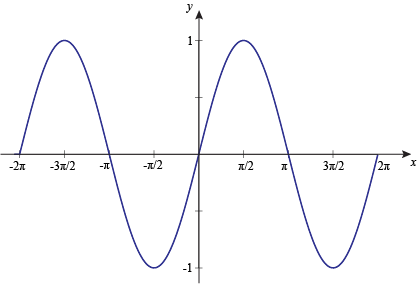Next we limit the domain to [-90°, 90°]. In radians, that's [-π2, π2].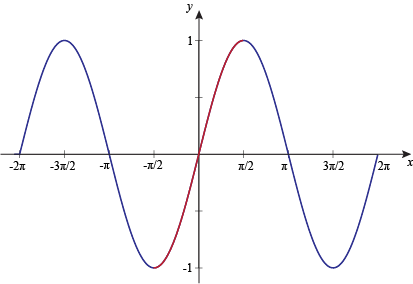To find the inverse sine graph, we need to swap the variables: x becomes y, and y becomes x. Here's the graph of the inverse sine function, y = sin-1 x (or y = arcsin x):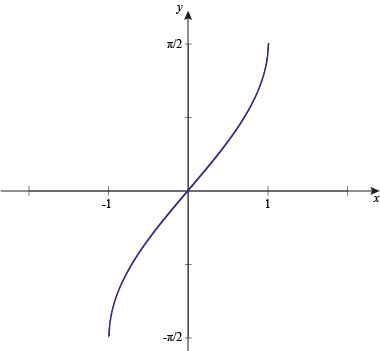Inverse sine has a domain of [-1, 1] and a range of [-π2, π2]. See how the angles are on the y-axis this time, instead of on the x-axis like they were for the sine function?

This means that when we're actually working problems using the inverse sine function, we'll be limited to Quadrant I and Quadrant IV (location is everything, as they say).

• ### Graph of Inverse Cosine Function

The graph of the inverse cosine function is a lot like the graph of the inverse sine function. They're tight like that. It needs to be chopped into pieces, too, in order to pass the vertical line test. Poor inverse trig functions. They never get to be themselves.

To graph the inverse cosine function, we limit or choose a section of our regular cosine graph to work with. Here's y = cos x.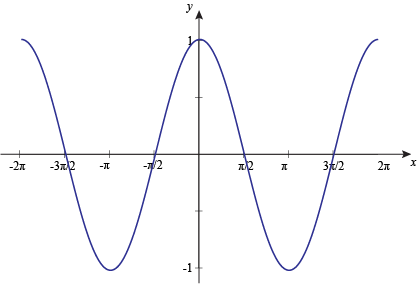Next, we limit the domain to [0°, 180°], or [0, π] if you're feeling radian-y.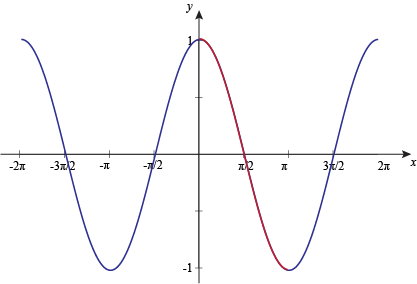To find the inverse cosine graph, we have to swap the variables, which gives us: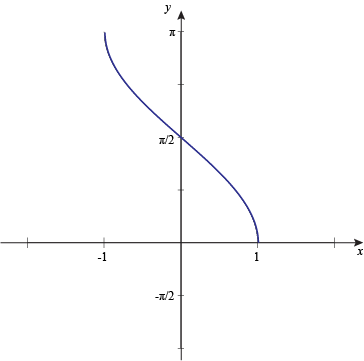Inverse cos has a domain of [-1, 1] and a range of [0°, 180°], or [0, π].

That limits us to Quadrant I and Quadrant II when we're solving problems with the inverse cosine function.

• ### Solving Inverse Trigonometric Equations

Let's take all this highfalutin knowledge and use it in some real, live problems. Otherwise, it's just hanging out at the mall all day spending money on handbags.

### Sample Problem

Find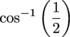.

First, we need to ask ourselves: where is cosine positive? (Is it near Waldo?)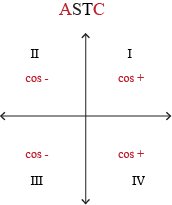Cosine is positive in Quadrants I and IV, but inverse cosine is limited to the range [0, π], so we're in Quadrant I.

Now we ask ourselves: for what angle is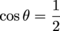?

Knowing your reference triangles will be really helpful here (along with an extra-large pizza, hold the anchovies).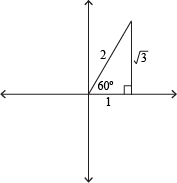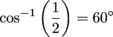### Sample Problem

Find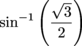.

Where is sine positive?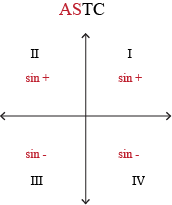Sine is positive in Quadrants I and II, but inverse sine is limited by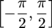. Therefore, we must be partying in Quadrant I. We ask ourselves: for what angle is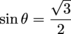?

Think: reference triangles.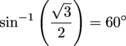### Sample Problem

Find tan-1(1).

Where is tangent positive?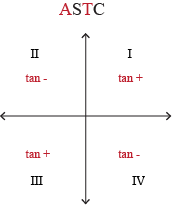Tangent is positive in Quadrants I and III, but inverse tangent is limited to the range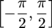. That's just how he rolls. We must be in Quadrant I.

Again, we ask ourselves: for what angle is tan θ = 1?

Reference triangles, anyone?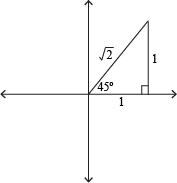tan-1(1) = 45°

### Sample Problem

Find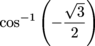.Cosine is negative in Quadrants II and III, but inverse cosine is limited to [0, π]. So our angle's gotta be in Quadrant II.

We ask ourselves: for what angle is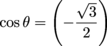? Man, we're sure asking ourselves a lot of questions.

Think: reference triangles (if you're tired of thinking, chill with "Angry Birds").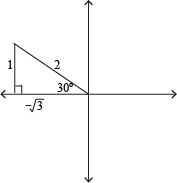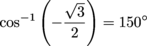If you're stuck on the 150°, here's what you can do. The reference angle is 30°, but we're in Quadrant II right now. That means we need to subtract our reference angle from 180° to get the actual angle.

180° – 30° = 150°

### Sample Problem

Find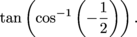Whoa. What we're really looking for is the tangent of the angle whose cosine is negative one-half. Let's work from the inside out. (This approach works in math, and maybe psychology.)

Solve the inside first. For what angle is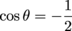?

Once again, it's back to those reference triangles, but now ask yourself that weighty question: where is cosine negative?But inverse cosine is bounded by [0, π], which means our angle is in Quadrant II.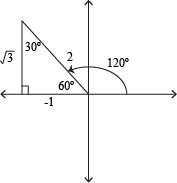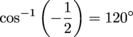Now we find tan(120°).Tangent is negative in Quadrant II, so we'll have a negative answer.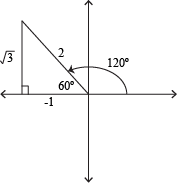So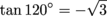.